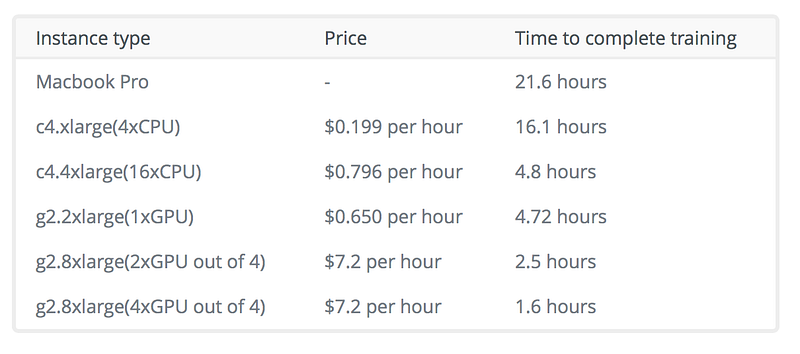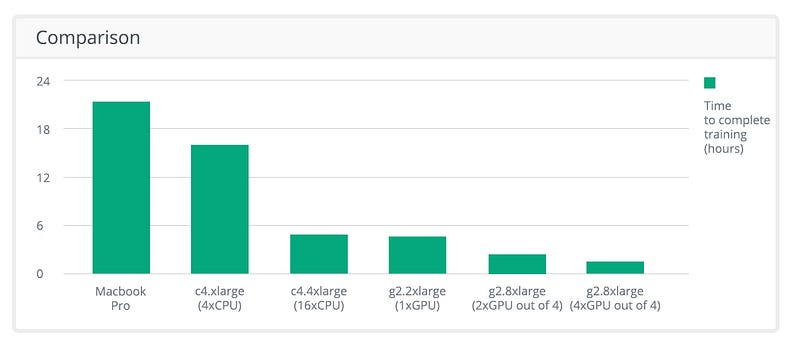# How to Train TensorFlow Models Using GPUs

### GPUs can accelerate the training of machine learning models. In this post, explore the setup of a GPU-enabled AWS instance to train a neural network in TensorFlow.

· AI Zone · Tutorial
Save
57.36K Views

In recent years, there has been significant progress in the field of machine learning. Much of this progress can be attributed to the increasing use of graphics processing units (GPUs) to accelerate the training of machine learning models. In particular, the extra computational power has lead to the popularization of deep learning — the use of complex, multi-level neural networks to create models, capable of feature detection from large amounts of unlabeled training data.

## Introduction to GPUs

GPUs are great for deep learning because the type of calculations they were designed to process are the same as those encountered in deep learning. Images, videos, and other graphics are represented as matrices so that when you perform any operation, such as a zoom-in effect or a camera rotation, all you are doing is applying some mathematical transformation to a matrix.

In practice, this means that GPUs, compared to central processing units (CPUs), are more specialized at performing matrix operations and several other types of advanced mathematical transformations. This makes deep learning algorithms run several times faster on a GPU compared to a CPU. Learning times can often be reduced from days to mere hours.

## GPUs in Machine Learning

So, how would one approach using GPUs for machine learning tasks? In this post, we will explore the setup of a GPU-enabled AWS instance to train a neural network in TensorFlow.

To start, create a new EC2 instance in the AWS control panel.

We will be using Ubuntu Server 16.04 LTS (HVM) as the OS, but the process should be similar on any 64-bit Linux distro.

For the instance type, select g2.2xlarge — these are enabled with the NVIDIA GRID GPU. There are also instances with several of these GPUs, but utilizing more than one requires additional setup which will be discussed later in this post.

Finish the setup with your preferred security settings.

Once the setup and creation are done, SSH into your instance.

Python should already be present on the system, so install the required libraries:

``````sudo apt-get update
sudo apt-get install python-pip python-dev``````

Next, install TensorFlow with GPU support enabled. The simplest way is:

``pip install tensorflow-gpu``

However, this might fail for some installations. If this happens, there is an alternative:

``````export
TF_BINARY_URL=https://storage.googleapis.com/tensorflow/linux/gpu/tensorflow_gpu-0.12.1-cp27-none-linux_x86_64.whl
sudo pip install --upgrade \$TF_BINARY_URL``````

If you get a `locale.Error: unsupported locale setting` during TF installations, enter:

``export LC_ALL=C``

Then, repeat the installation process.

If no further errors occur, the TF installation is over. However, for GPU acceleration to properly work, we still have to install Cuda Toolkit and cuDNN.

First, let's install the Cuda Toolkit.

Before you start, please note that the installation process will download around 3 GB of data.

``````wget "http://developer.download.nvidia.com/compute/cuda/repos/ubuntu1604/x86_64/cuda-repo-ubuntu1604_8.0.44-1_amd64.deb"
sudo dpkg -i cuda-repo-ubuntu1604_8.0.44-1_amd64.deb
sudo apt-get update
sudo apt-get install cuda``````

Once the CUDA Toolkit is installed, download cuDNN Library for Linux (note that you will need to register for the Accelerated Computing Developer Program) and copy it to your EC2 instance.

``````sudo tar -xvf cudnn-8.0-linux-x64-v5.1.tgz -C /usr/local
export PATH=/usr/local/cuda/bin:\$PATH
export LD_LIBRARY_PATH="\$LD_LIBRARY_PATH:/usr/local/cuda/lib64:/usr/local/cuda/extras/CUPTI/lib64"
export CUDA_HOME=/usr/local/cuda``````

Finally, the setup process is over and we can test the installation:

``````python
>>> import tensorflow as tf
>>> sess = tf.Session()``````

You should see `Found device 0 with properties: name: GRID K520`:

``````>>> hello_world = tf.constant("Hello, world!")
>>> print sess.run(hello_world)``````

`Hello, world!` will be displayed:

``>>> print sess.run(tf.constant(123)*tf.constant(456))``

`56088` is the correct answer.

The system is now ready to utilize a GPU with TensorFlow.

The changes to your TensorFlow code should be minimal. If a TensorFlow operation has both CPU and GPU implementations, the GPU devices will be prioritized when the operation is assigned to a device.

If you would like a particular operation to run on a device of your choice instead of using the defaults, you can use `with tf.device` to create a device context. This forces all the operations within that context to have the same device assignment.

``````# Creates a graph.
with tf.device('/gpu:0'):
a = tf.constant([1.0, 2.0, 3.0, 4.0, 5.0, 6.0], shape=[2, 3], name='a')
b = tf.constant([1.0, 2.0, 3.0, 4.0, 5.0, 6.0], shape=[3, 2], name='b')
c = tf.matmul(a, b)
# Creates a session with log_device_placement set to True.
sess = tf.Session(config=tf.ConfigProto(log_device_placement=True))
# Runs the op.
print sess.run©``````

If you would like to run TensorFlow on multiple GPUs, it is possible to construct a model in a multi-tower fashion and assign each tower to a different GPU. For example:

``````# Creates a graph.
c = []
for d in ['/gpu:2', '/gpu:3']:
with tf.device(d):
a = tf.constant([1.0, 2.0, 3.0, 4.0, 5.0, 6.0], shape=[2, 3])
b = tf.constant([1.0, 2.0, 3.0, 4.0, 5.0, 6.0], shape=[3, 2])
c.append(tf.matmul(a, b))
with tf.device('/cpu:0'):
sum = tf.add_n(c)
# Creates a session with log_device_placement set to True.
sess = tf.Session(config=tf.ConfigProto(log_device_placement=True))
# Runs the op.
print sess.run(sum)``````

## Benefits of Utilizing the GPU

For benchmarking purposes, we will use a convolutional neural network (CNN) for recognizing images that are provided as part of the TensorFlow tutorials. CIFAR-10 classification is a common benchmark problem in machine learning. The task is to classify RGB 32x32 pixel images across 10 categories.

Let’s compare the performance of training this model on several popular configurations:## Results

As demonstrated by the results, in this specific example, it takes the power of 16 CPUs to match the power of 1 GPU. At the time of writing, utilizing a GPU is also 18% cheaper for the same training time.

If you enjoyed this article and want to learn more about TensorFlow, check out this collection of tutorials and articles on all things TensorFlow.

Topics:
tensorflow, gpu, deep learning, machine learning, ai, tutorial, training data, neural network

Published at DZone with permission of Kseniya Savitsina.

Opinions expressed by DZone contributors are their own.

Comments